## Calculate Percentage in Excel – with 7 Formula Examples

Calculating percentage is useful in many areas of life, for example, calculating the discount price or the percentage of total. In this tutorial, it provides some examples and formulas to tell you how to calculate percentages in Excel.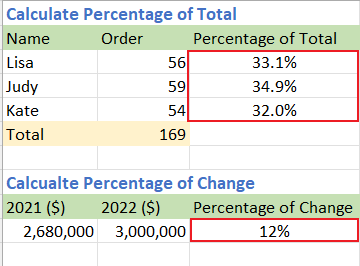### Get the percentage of total

#### Example 1: Get the percentage of a given total

For example, you have some values in column B (B2:B4), and the total number of these values is in cell B5 as below screenshot shown: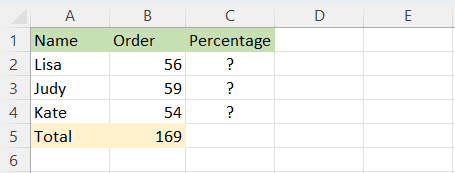Now you want to get the percentage of each value of the total, please do as below:

##### Step 1: Use formula to get the percentage of each value of the total

1. Select a blank cell, here is C2, type below formula, then press Enter key to get the first percentage:

``=B2/\$B\$5``
Note: To make the denominator an absolute reference, either type the dollar sign (\$) manually or click the cell reference in formula bar and press F4 key.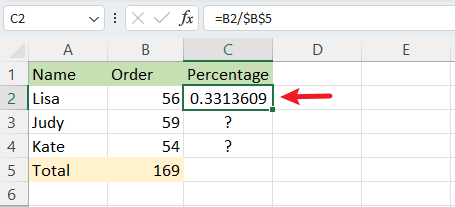2. Then double click at the auto fill handle (the small green square in the right corner of the formula result cell) to fill the formula to below cells.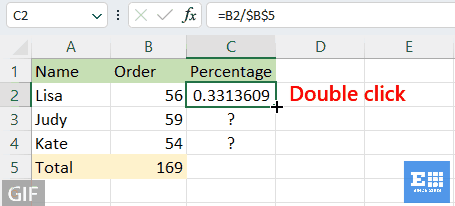##### Step 2: Format result as percentage

Select the result cells, then click Home tab, and go to Number group, select Percentage Style. (Or you can use shortcuts Shift + Ctrl + % to format cells as percentage.)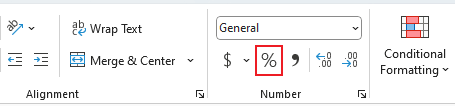Now the results are shown in percentage formatting.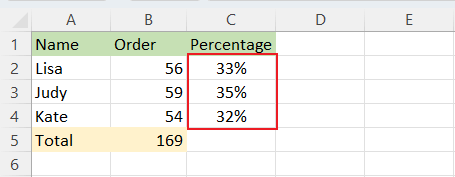Note: You can change the decimal number by clicking Increase Decimal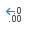or Decrease Decimal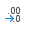in Number group under Home tab.

#### Example 2: Get the percentage of an unknown total

For example, there is a table that contains the scores of students. Now you want to get the percentage of score 1 of each total: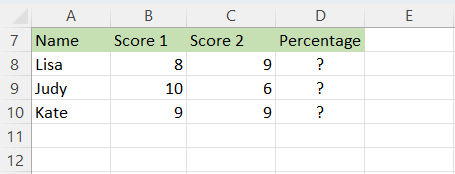##### Step 1: Use formula to get the percentage of each value of the total

1. Select a blank cell, here is D8, type below formula, then press Enter key to get the first percentage:

``=B8/SUM(B8:C8)``
NoteSUM function is used to get the total of numbers. SUM(B8:C8) gets the total scores of Lisa.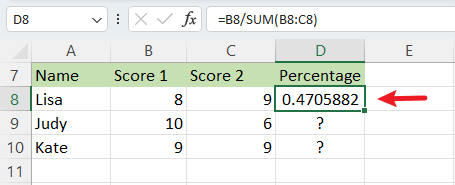2. Then double click at the auto fill handle (the small green square in the right corner of the formula result cell) to fill the formula to below cells.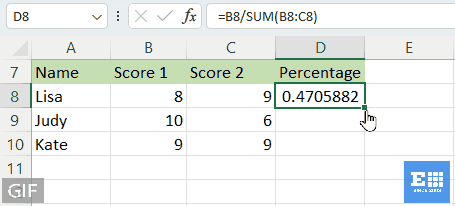##### Step 2: Format result as percentage

Select the result cells, then click Home tab, and go to Number group, select Percentage Style. (Or you can use shortcuts Shift + Ctrl + % to format cells as percentage.)Now the results are shown in percentage formatting.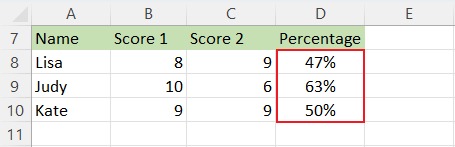Note: You can change the decimal number by clicking Increase Decimalor Decrease Decimalin Number group under Home tab.

### Get the percentage of change between two numbers

Let’s say the income of company A in year 2022 is \$3,000,000, and the income of company A in year 2021 is \$2,680,000, what is the percentage of change between these two years? You can calculate the difference by subtracting the new income (2022) to the original income (2021), then dividing the result by the original income (2021).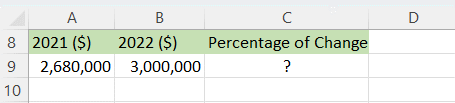##### Step 1: Use formula to get the percentage of each value of the total

Select a blank cell, here is C9, type below formula, then press Enter key to get the percentage of change:

``=(B9-A9)/A9``
Note: Operation in parentheses will be calculated first. Cell A9 contains the older price (2021), Cell B9 contains the newer price (2022).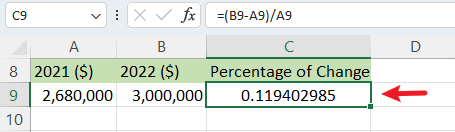If you want to apply this formula to below cells, double click at the auto fill handle (the small green square in the right corner of the formula result cell) to fill.

##### Step 2: Format result as percentage

Select the result cell, then click Home tab, and go to Number group, select Percentage Style. (Or you can use shortcuts Shift + Ctrl + % to format cells as percentage.)Now the result is shown in percentage formatting.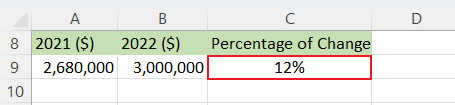Notes:
1. If the difference result is positive, which means that the last value is increased compare to the first one. If the result is negative, which means that the last value is decreased compare to the first one.

2. You can change the decimal number by clicking Increase Decimalor Decrease Decimalin Number group under Home tab.

3. In the formula, if the divisor (the older value) is zero or blank, the formula will return #DIV/0! error value. For avoiding this, you can use formula:

``=IF(A9=0,1,(B9-A9)/A9)``

Then the result is returned 100%.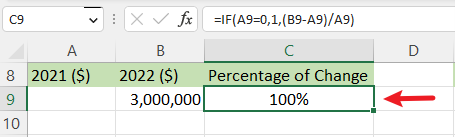4. If you do not want to format the result as percentage, you can multiply the result by 100: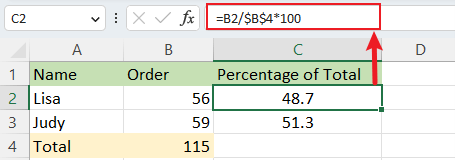### Increase or decrease a number by a percentage

#### Example 1: Increase a number by a percentage

Let’s say that your annual salary is \$320000 last year, your company decides to increase your annual salary by 20% this year, how much you will earn this year?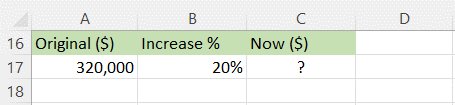Select a blank cell, here is C17, type below formula, then press Enter key:

``=A17*(1+B17)``
Note: Operation in parentheses will be calculated firstly. Cell A17 contains the original number, cell B17 contains the increase percentage.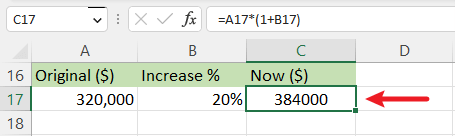#### Example 2: Decrease a number by a percentage

Suppose the manager wants to decrease the advertising cost of \$10,000 by 25% next month, what is the new monthly advertising cost?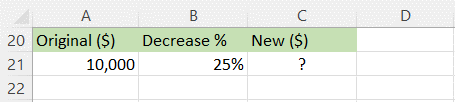Select a blank cell, here is C21, type below formula, then press Enter key:

``=A21*(1-B21)``
Note: Operation in parentheses will be calculated firstly. Cell A21 contains the original number, cell B21 contains the decrease percentage.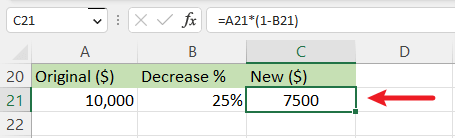### Get the total by given amount and percentage

Suppose the sale price of a laptop is \$120, which is 20% off the original price. The question is what is the original price of this laptop?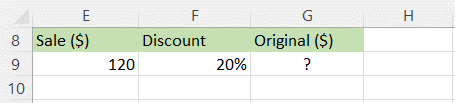Select a blank cell, here is G9, type below formula, then press Enter key to get the total number:

``=E9/(1-F9)``
Note: Operation in parentheses will be calculated first. Cell E9 contains the sale price, cell F9 contains the discount percentage.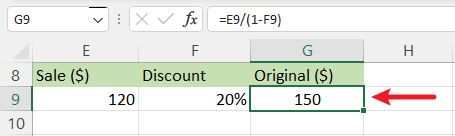### Get the amount by given total and percentage

If the original price of a coat is \$110, but you need to pay additional 12% in sales tax, how much you need to pay for the sales tax?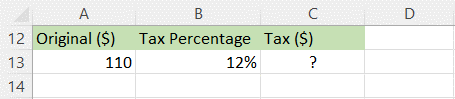Select a blank cell, here is C13, type below formula, then press Enter key:

``=A13*B13``
Note: Cell A13 contains the price, cell B13 contains the tax percentage.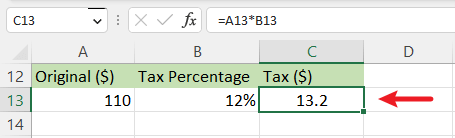### Best Office Productivity Tools

 Popular Features: Find, Highlight or Identify Duplicates   |  Delete Blank Rows   |  Combine Columns or Cells without Losing Data   |   Round without Formula ... Super Lookup: Multiple Criteria VLookup  |   Multiple Value VLookup  |   VLookup Across Multiple Sheets   |   Fuzzy Lookup .... Advanced Drop-down List: Quickly Create Drop Down List   |  Dependent Drop Down List   |  Multi-select Drop Down List .... Column Manager: Add a Specific Number of Columns  |  Move Columns  |  Toggle Visibility Status of Hidden Columns  |  Compare Ranges & Columns ... Featured Features: Grid Focus   |  Design View   |   Big Formula Bar   |  Workbook & Sheet Manager   |  Resource Library (Auto Text)   |  Date Picker   |  Combine Worksheets   |  Encrypt/Decrypt Cells   |  Send Emails by List   |  Super Filter   |   Special Filter (filter bold/italic/strikethrough...) ... Top 15 Toolsets:  12 Text Tools (Add Text, Remove Characters, ...)   |   50+ Chart Types (Gantt Chart, ...)   |   40+ Practical Formulas (Calculate age based on birthday, ...)   |   19 Insertion Tools (Insert QR Code, Insert Picture from Path, ...)   |   12 Conversion Tools (Numbers to Words, Currency Conversion, ...)   |   7 Merge & Split Tools (Advanced Combine Rows, Split Cells, ...)   |   ... and more

Supercharge Your Excel Skills with Kutools for Excel, and Experience Efficiency Like Never Before. Kutools for Excel Offers Over 300 Advanced Features to Boost Productivity and Save Time.  Click Here to Get The Feature You Need The Most...#### Office Tab Brings Tabbed interface to Office, and Make Your Work Much Easier

• Enable tabbed editing and reading in Word, Excel, PowerPoint, Publisher, Access, Visio and Project.
• Open and create multiple documents in new tabs of the same window, rather than in new windows.
• Increases your productivity by 50%, and reduces hundreds of mouse clicks for you every day!